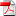# A Principal-Agent Problem for Emissions' Reduction

1
Juin

RR-FiME-12-06

### Giuseppe Benedetti

We analyze a principal-agent problem between the state (principal) and a firm (agent) which produces carbon emissions. In particular, the aim of the state is to motivate the firm to reduce those emissions as much as possible by structuring an appropriate incentive policy. We allow for two different kinds of incentives: a \negative" one, typically represented by a fee to pay at a given time T if emissions are too high; and a \positive" one, in the form of continuous-time payments to the agent. Given an incentive policy, we solve the agent's problem using the stochastic maximum principle to derive alternative representations of the optimal effort in terms of Backward Stochastic Differential Equations (BSDEs), and showing uniqueness of the optimal effort up a certain class of policies. This also allows to prove that the agent's utility is (strictly) increasing with respect to incentives and to discuss the sensitivity of the optimal effort when risk aversion or emissions' volatility change. Under some regularity hypotheses, the problem boils down to solving a certain nonlinear PDE, for which we give a suitable discretization scheme. We then perform some numerical experiments to show how the agent behaves in two particular cases: in line with intuition, the optimal e ort is bell-shaped in the case of a forfeitary fee while it exhibits a monotone behavior in presence of a linearly increasing fee. The last section is devoted to the problem of the principal, who needs to propose an incentive plan by taking into account the subsequent agent's behaviour. We show that under proper assumptions the problem is similar to the agent's one and can therefore be solved using the same techniques. Again we provide a numerical example to illustrate in particular the optimal choice of continuous-time incentives.

•rr-fime-12-06